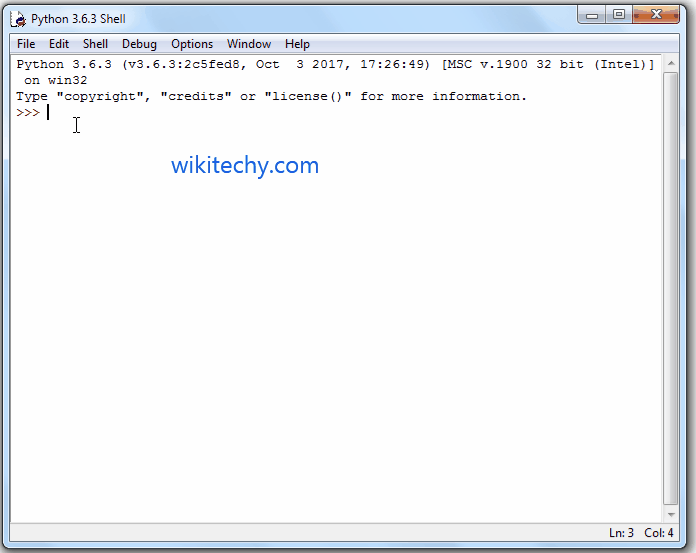# python tutorial - Python Program to find Volume and Surface Area of a Cone - learn python - python programming

• To write Python Program to find Volume and Surface Area of a Cone with example.
• Before we step into the program, Let see the definitions and formulas behind Surface area of a Cone and Volume of a ConeLearn Python - Python tutorial - Python Program to find Volume and Surface Area of a Cone - Python examples - Python programs

## Surface Area of a Cone

• If we know the radius and Slant of a Cone then we calculate the Surface Area of Cone using the below formula:
• If we know the radius and height of a Cone then we calculate the Surface Area of Cone using the below formula:
• Because radius, height and Slant make the shape as right-angled Triangle. So, Using the Pythagoras theorem:

## Volume of a Cone

• The amount of space inside the Cone is called as Volume.
• If we know the radius and height of the Cone then we can calculate the Volume using the formula:

## Python Program to find Volume and Surface Area of a Cone

• This program allows user to enter the value of a radius and height of a Cone.
• Using these values it will calculate the Surface Area, Volume, length of a side (Slant) and Lateral Surface Area of a Cone as per the formulas.

## Analysis

• First, We imported the math library using the following statement.
• This will allow us to use the mathematical functions like math.pi and math.sqrt.
• If you fail to include this line then math.pi will through an error.
• Below statements will ask the user to enter radius and height values and it will assign the user input values to respected variables.
• Such as first value will be assigned to radius and second value to height
• Next, We are calculating Volume, Surface Area, Lateral Surface Area and Length of a Side (Slant) of a Cone using their respective Formulas:
• Following print statements will help us to print the Volume and Surface area of a Cube

## OutputLearn Python - Python tutorial - Python Program to find Volume and Surface Area of a Cone - Python examples - Python programs

• We have entered the Radius of a Cone = 5 and Height = 12

As per the Pythagoras theorem, We can calculate the Slant (Length of a side):
l² = h² + r²
l = √h² + r²
l = √12² + 5²
l = √144 + 25
l = √169
l = 13

The Surface Area of a Cone is
Surface Area of a Cone = πr² +πrl
Surface Area of a Cone = πr (r + l)
Surface Area of a Cone = math.pi * radius * (radius + l)
Surface Area of a Cone = 3.14 * 5 * ( 5 +13)
Surface Area of a Cone = 3.14 * 5 * 18
Surface Area of a Cone = 282.6

The Volume of a Cone is
Volume of a Cone = 1/3 πr²h
Volume of a Cone = (1.0/3) * math.pi * radius * radius * height
Volume of a Cone = (1.0/3) * 3.14 * 5 * 5 * 12;
Volume of a Cone = 314

The Lateral Surface Area of a Cone is
Lateral Surface Area = πrl
Lateral Surface Area = math.pi * radius * l
Lateral Surface Area = 3.14 * 5 * 13
Lateral Surface Area = 204.1

Let us calculate the Radius of a Cone using the radius without using the Slant (Standard Formula):
Surface Area of a Cone = πr² +πr √h² + r²
Surface Area of a Cone = πr (r + √h² + r²)
Surface Area of a Cone = 3.14 * 5 * ( 5 + √12² + 5²)
Surface Area of a Cone = 3.14 * 5 * ( 5 + √169)
Surface Area of a Cone = 3.14 * 5 * ( 5 + 13)
Surface Area of a Cone = 3.14 * 5 * 18
Surface Area of a Cone = 282.6

## Python Program to find Volume and Surface Area of a Cone using functions

• This program allows user to enter the value of a radius and height of a Cone.
• We will pass the radius and height values to the function argument and then it will calculate the Surface Area and Volume of a Cone as per the formula.

## Analysis:

• First, We defined the function with two argument using def keyword.
• It means, User will enter the radius and height of a Cone.
• Using those values, above function will calculate the Surface Area and Volume of a Sphere as we explained in first example

## OutputLearn Python - Python tutorial - Python Program to find Volume and Surface Area of a Cone using functions - Python examples - Python programs

NOTE: We can call the function with arguments in .py file directly or else we can call it from the python shell. Please don’t forget the function arguments